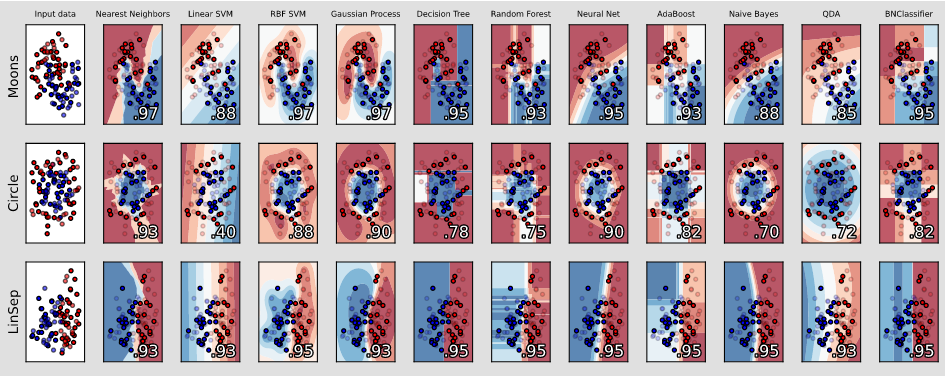# Classifier

Probabilistic classification in pyAgrum aims to propose a scikit-learn-like (binary and multi-class) classifier class that can be used in the same codes as scikit-learn classifiers. Moreover, even if the classifier wraps a full Bayesian network, skbn optimally encodes the classifier using the smallest set of needed features following the d-separation criterion (Markov Blanket).An example from scikit-learn where a last column with a BNClassifier has been added flawlessly (see this notebooks).

The module proposes to wrap the pyAgrum’s learning algorithms and some others (naive Bayes, TAN, Chow-Liu tree) in the fit method of a classifier. In order to be used with continuous variable, the module proposes also some different discretization methods.

skbn is a set of pure python3 scripts based on pyAgrum’s tools.

Tutorials

Notebooks on scikit-learn compliant classifiers in pyAgrum,

Reference